Example Questions

2 Next →

Example Question #11 : Sales Tax

Todd is buying a laptop that is discounted by 20%. Included in the amount he pays is a 7% sales tax on the discounted price. If the original price of the laptop is $995 (before the discount) what is the total amount that Todd pays, rounded to the nearest cent? Possible Answers: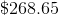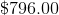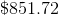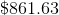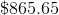Correct answer:Explanation: If a$995 computer is discounted by 20%, that means its new price is 80% of its original price.

We can find the new price by multiplying $995 by .8, which gives us$796.

If Todd must also pay a 7% sales on the discounted price, we can find his total amount by multiplying $796 by 1.07, which gives us$851.72.

2 Next →